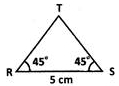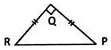# CBSEtips.in

## Thursday, 25 February 2021

### CBSE Class 7 Maths - MCQ and Online Tests - Unit 10 - Practical Geometry

#### CBSE Class 7 Maths – MCQ and Online Tests – Unit 10 – Practical Geometry

Every year CBSE students attend Annual Assessment exams for 6,7,8,9,11th standards. These exams are very competitive to all the students. So our website provides online tests for all the 6,7,8,9,11th standards’ subjects. These tests are also very effective and useful for those who preparing for any competitive exams like Olympiad etc. It can boost their preparation level and confidence level by attempting these chapter wise online tests.

These online tests are based on latest CBSE syllabus. While attempting these, our students can identify their weak lessons and continuously practice those lessons for attaining high marks. It also helps to revise the NCERT textbooks thoroughly

#### CBSE Class 7 Maths – MCQ and Online Tests – Unit 10 – Practical Geometry

Question 1.
Study the steps of construction given.
Step 1: Draw a ray OA.
Step 2: With O as centre and any convenient radius draw an arc MN to cut OA at M.
Step 3: With M as centre and the same radius draw an arc to cut MN at P.
Step 4: With P as centre and the same radius, draw an arc to cut MN at Q.
Step 5: Draw OQ and produce it to D. An angle AOD is constructed.
What is the measure of ?AOD?
(a) 60°
(b) 30°
(c) 120°
(d) 45°

Hint:
The given steps of construction are to construct an angle of 120°.Question 2.
In ?XYZ, x, y and z denote the three sides. Which of the following is incorrect’?
(a) x – y > z
(b) x + z > y
(c) x – y < z
(d) x + y > z

Answer: (a) x – y > z
Hint:
The difference of two sides of a triangle is less than its third side.

Question 3.
The idea of equal alternate angles is used to construct which of the following?
(a) A line parallel to a given line
(b) A triangle
(c) A square
(d) Two triangles

Answer: (a) A line parallel to a given line.

Question 4.
A Given AB = 3 cm, AC = 5 cm,and ?B = 30°, ?ABC cannot be uniquely constructed, with AC as base, why?
(a) Two sides and included angle are given.
(b) The other two angles are not given.
(c) The vertex B cannot be uniquely located.
(d) The vertex A coincides with the vertex C.

Answer: (c) The vertex B cannot be uniquely located.

Question 5.
A line panda point X not on it are given. Which of the following is used to draw a line parallel to p through X?
(a) Equal corresponding angles.
(b) Congruent triangles.
(c) Angle sum property of triangles.
(d) Pythagoras’ theorem.

Answer: (a) Equal corresponding angles.
Hint:
Corresponding angles of parallel lines are equal.

Question 6.
? PQR is such that ?P = ?Q = ?R = 60° which of the following is true?
(a) ? PQR is equilateral.
(b) ? PQR is acute angled.
(c) Both [a] and [b]
(d) Neither [a] nor [b]

Answer: (c) Both [a] and [b]
Hint:
In ?PQR since all the angles are acute, it is acute angled. Also since all the angles are equal, it is equilateral.

Question 7.
Which vertex of ?ABC is right angled if $$\overline { AB }$$ = 8 cm, $$\overline { AC }$$ = 6 cm,and $$\overline { BC }$$ = 10 cm,?
(a) ?C
(b) ?A
(c) ?B
(d) A or C

Hint:
From the given measurements, $$\overline { BC }$$ is the hypotenuse. The angle opposite to $$\overline { BC }$$ is ?A which is a right angle.

Question 8.
In ?RST, R = 5 cm, and ?SRT = 45° and ?RST = 45°. Which criterion can be used to construct ?RST?
(a) A.S.A. criterion
(b) S.A.S. criterion
(c) S.S.S. criterion
(d) R.H.S. criterion

Answer: (a) A.S.A. criterion
Hint:Clearly, from the figure two angles and the included side are given. So, A.S.A. criterion can be used to construct ARST.

Question 9.
Identify the criterion of construction of the equilateral triangle LMN given LM = 6 cm.
(a) S.A.S. criterion
(b) R.H.S. criterion
(c) A.S.A. criterion
(d) S.S.S. criterion

Answer: (d) S.S.S. criterion
Hint:
Since ALMN is equilateral the measurement of one side is used for the other two sides of the triangle. Hence ALMN can be constructed by S.S.S. criterion.

Question 10.
How many perpendicular lines can be drawn to a line from a point not on it?
(a) 1
(b) 2
(c) 0
(d) Infinite

Hint:As can be seen from the given figure, one and only one perpendicular line can be drawn to a given line from a point not on it.

Question 11.
Identify the false statement.
(a) A triangle with three equal sides is called an equilateral triangle.
(b) A triangle with a right angle is called a right angled triangle.
(c) A triangle with two equal sides is called a scalene triangle.
(d) A right angled triangle has two acute angles and a right angle.

Answer: (c) A triangle with two equal sides is called a scalene triangle.

Question 12.
?PQR is constructed such that PQ = 5 cm, PR = 5 cm and ?RPQ = 50° Identify the type of triangle constructed.
(a) An isosceles triangle
(b) An acute angled triangle
(c) An obtuse angled triangle
(d) Both [a] and [b]

Answer: (d) Both [a] and [b]

Question 13.
Which of the following is NOT constructed using a ruler and a set square?
(a) A perpendicular to a line from a point not on it.
(b) A perpendicular bisector of a line segment.
(c) A perpendicular to a line at a point on the line.
(d) A line parallel to a given line through a given point.

Answer: (b) A perpendicular bisector of a line segment.

Question 14.
Which of the following statements is incorrect?
(a) The sum of angles in a triangle is 2 right angles.
(b) The exterior angle of a triangle is equal to the interior angle of the triangle.
(c) The hypotenuse is the longest side of a right angled triangle.
(d) All the above.

Answer: (b) The exterior angle of a triangle is equal to the interior angle of the triangle.

Question 15.
A triangular sign board is isosceles. If the unequal side is 7 cm and one of the equal sides is 6 cm, what is the measure of the third side?
(a) 5 cm
(b) 6 cm
(c) 7 cm
(d) Either [a] or [c]

Answer: (b) 6 cm

Question 16.
In the given figure, find the measure of ?ROT, if PQ = QR and ?QPR = 60°.(a) 60°
(b) 140°
(c) 120°
(d) 100°

Hint:
?PQR is isosceles since PQ = QR.
? ?QPR = ?QRP = 60° ?RQT is the exterior angle of ?PQR which is equal to the sum of interior opposite angles ?P and ?R.
Hence, ?RQT = 60° + 60° = 120°.

Question 17.
Which among the following is used to construct a triangle?
(a) The lengths of the three sides.
(b) The perimeter of the triangle.
(c) The measures of three angles.
(d) The names of three vertices.

Answer: (a) The lengths of the three sides.
Hint:
S.S.S. criterion can be used indirectly to construct a triangle given the lengths of its three sides.

Question 18.
In which of the following cases can a triangle be constructed?
(a) Measures of three sides are given.
(b) Measures of two sides and an included angle are given.
(c) Measures of two angles and the side between them are given.
(d) All the above.

Answer: (d) All the above.

Question 19.
Based on the sides of a triangle, which of the following is a classification of triangles?
(a) A right angled triangle
(b) An acute angled triangle
(c) An obtuse angled triangle
(d) An isosceles triangle

Answer: (d) An isosceles triangle

Question 20.
Which of the following is used to draw a line parallel to a given line?
(a) A protractor
(b) A set square
(c) A ruler
(d) A ruler and compasses

Answer: (d) A ruler and compasses
Hint:
A line parallel to a given line can be drawn using a ruler and a compass.

Question 21.
Direction: David folds a sheet of paper. The dotted lines as shown in the figure are the creases formed, which are named as l, m and n.What can you say about lines I and n?
(a) l // n
(b) l ? n
(c) I is the same line as n
(d) Neither [a] nor [b]

Answer: (b) l ? n
Hint:
A 90° angle is formed at the intersection of l and n. So l ? n.

Question 22.
A Choose the correct option in which a triangle CANNOT be constructed with the given lengths of sides.
(a) 3 cm, 4 cm, 5 cm
(b) 7 cm, 6 cm, 5 cm
(c) 10 cm, 7 cm, 2 cm
(d) 12 cm, 8 cm, 6 cm

Answer: (c) 10 cm, 7 cm, 2 cm
Hint:
The difference of any two sides of a triangle must be less than the third side. This property of triangles is not satisfied by the given measurements as 10 – 7 = 3 > 2 and 10 – 2 = 8 > 7, though 7 – 2 = 5 < 10 is true.

Question 23.
Identify the true statement.
(a) A triangle with 3 equal sides is isosceles.
(b) A triangle with a 110° angle is right angled.
(c) A triangle with 3 acute angles is acute angled.
(d) A triangle with 2 equal sides is equilateral.

Answer: (c) A triangle with 3 acute angles is acute angled.

Question 24.
An isosceles triangle is constructed as shown in the figure.Which of the given statements is incorrect?
(a) $$\overline { PR }$$ is the hypotenuse of ?PQR.
(b) ?PQR is an equilateral triangle.
(c) ?PQR is a right angled triangle.
(d) If right angled ?PQR has its equal angles measuring 45° each.

Answer: (b) ?PQR is an equilateral triangle.

Question 25.
?PQR is constructed with all its angles measuring 60° each. Which of the following is correct?
(a) ?PQR is an equilateral triangle.
(b) ?PQR is isosceles triangle.
(c) ?PQR is a scalene triangle.
(d) ?PQR is a right angled triangle.

Answer: (a) ?PQR is an equilateral triangle.

Share: